# How to implement quick sort in JavaScript?

In this article, we are going to discuss how to implement quick sort in JavaScript with suitable examples.

## Quick sort

The Quick sort is a divide and conquers algorithm similar to the merge sort. In this, we pick a pivot element and divide the array around the pivot element. There are many ways to pick the pivot element.

• Always pick the first element as a pivot element.

• Always pick the last element as a pivot element. (Implemented in the below program)

• Pick a random element as pivot element

• Pick the middle element as pivot element.

The main process in quick sort is partition. The aim of partition is, given an array and consider an element x in the array as pivot element. Keep the pivot element at the correct position in sorted array. Then put all the elements which are smaller than the pivot element in left side and the elements which are greater than the pivot element will be on the right side.

### Input and Output Scenarios

Consider an array having some elements in it in a random order which are not sorted, we can sort the elements by performing merge sort. Let’s check the scenario below.

Input = [0, 10, 4, 1, 3];
Output = 0, 1, 3, 4, 10


### Simple Partition and Quick Sort Function

Selecting an element x as pivot. The elements which are smaller than pivot element will be on left side of pivot element and the elements which are greater than pivot element will be on right side of pivot element.

#### How quick sort works?

To know how the quick sort is working, let’s assume an array arr=[7, 4, 10, 6, 3, 9]

• Indexes are 0 1 2 3 4 5

• Low = 0, High = 5 and pivot element = 9

• Initially the index of smaller element, i = -1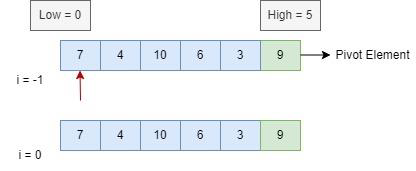• By comparing the first element to the pivot element and 7 is less than 9. So, 7 is placed at index 0 and i moved to i=0.

• Now, let’s compare 4 with pivot element and 4 is less than 9, So, i will be at i=1 and 4 will be placed there.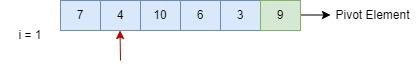• Compare 10 with pivot and 10 is greater than 9. i will not increment ( i = 1).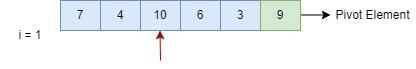• Let’s move to the next element 6, as 6 is smaller than pivot it will be in i=2. 9 will be in moved to i = 3.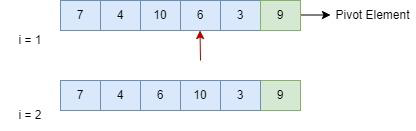• Comparing 3 with pivot and 3 is less than 10. So i will increment and become i=3 and 10 and 3 will be swapped.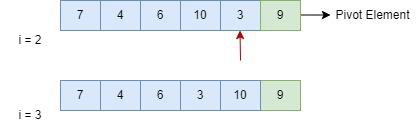• Now compare 10 with pivot. As 10 is greater pivot element it will be placed in i=4 and 10 will be in i=5.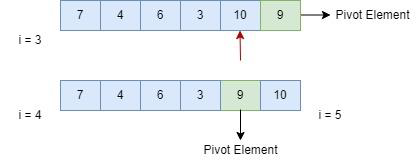### Partition

Now, let’s perform partition around pivot element.

• Pivot = 9.

• Perform the partition at 9, elements less than 9 should be on left and greater 9 should be right.

• Then consider the last element as pivot and continue the partition as shown in the figure below.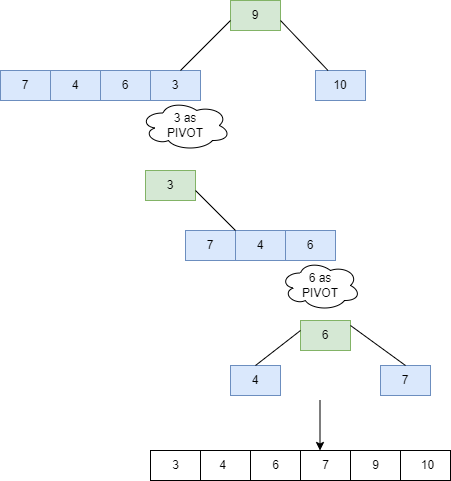Now, let’s implement the above process of quick sort in the following program.

### Example

In the following example, we are considering the last element as the pivot –

<!DOCTYPE html>
<html>
<title>Implementation of Quick Sort</title>
<body>
<script>
function Quicksort(array){
if (array.length < 2){
return array;
}
let pivot_element = array[array.length - 1]
let left_sub_array = [];
let right_sub_array = [];
for (let i = 0; i < array.length - 1; i++){
if (array[i] < pivot_element) {
left_sub_array.push(array[i])
} else {
right_sub_array.push(array[i])
}
}
return [...Quicksort(left_sub_array), pivot_element, ...Quicksort(right_sub_array)];
}
const array = [0, 10, 4, 1, 3];
document.write(Quicksort(array));
</script>
</body>
</html>